﻿ 1979年~2018年青藏高原气温与湿度特征分析 Temperature and Humidity Characteristics of the Qinghai-Tibet Plateau from 1979 to 2018

Climate Change Research Letters
Vol. 09  No. 04 ( 2020 ), Article ID: 36655 , 15 pages
10.12677/CCRL.2020.94038

Temperature and Humidity Characteristics of the Qinghai-Tibet Plateau from 1979 to 2018

Yueqi Li, Ruomei Zhong

School of Atmospheric Sciences, Chengdu University of Information Technology, Chengdu Sichuan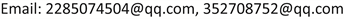Received: Jul. 2nd, 2020; accepted: Jul. 16th, 2020; published: Jul. 23rd, 2020ABSTRACT

This article will use the Chinese Meteorological Forced Data Set (CMFD) to analyze the characteristics of air temperature and humidity in the Qinghai-Tibet Plateau from 1979 to 2018, mainly to study the spatial and temporal distribution characteristics of air temperature and humidity from 1979 to 2018, as well as air temperature, humidity and precipitation, correlation of meteorological factors such as radiation. The conclusions are as follows: 1) The changes in air temperature and humidity in time series are consistent, but the change in humidity is greater than that in air temperature; the distribution of air temperature and humidity are affected by the terrain, and the annual average air temperature and specific humidity are averaged in the radial and latitude directions both have a great negative correlation with the altitude, that is, the temperature and humidity decrease with the increase of altitude; 2) In addition to the influence of natural factors such as altitude and latitude and longitude, temperature and humidity are also highly related to meteorological elements such as long-wave downward radiation and precipitation.

Keywords:The Tibetan Plateau, The Temperature, Humidity, The Correlation1979年~2018年青藏高原气温与 湿度特征分析1. 引言

2. 资料与方法

2.1. 研究区概况

2.2. 资料概括

2.3. 研究方法Figure 1. Topographic map of the Tibetan Plateau

1) 青藏高原区域平均下月累计降水的计算

$sum{A}_{j}=\underset{n=sum+1}{\overset{sum+ai}{\sum }}\stackrel{¯}{{A}_{n}},\left(j=1,2,3,\cdots ,12\right)$

2) 任意两个气象要素的相关系数计算

${r}_{\left(k,l\right)}=\frac{{s}_{kl}}{{s}_{k}{s}_{l}}=\frac{\underset{i=1}{\overset{n}{\sum }}{x}_{ki}{x}_{li}-n\stackrel{¯}{{x}_{k}}\stackrel{¯}{{x}_{l}}}{\sqrt{\underset{i=1}{\overset{n}{\sum }}{x}_{li}^{2}-n{\left(\stackrel{¯}{{x}_{i}}\right)}^{2}}\sqrt{\underset{i=1}{\overset{n}{\sum }}{x}_{ki}^{2}-n{\left(\stackrel{¯}{{x}_{i}}\right)}^{2}}}$

$t=\sqrt{n-2}\frac{r}{\sqrt{1-{r}^{2}}}$

3) 各气象要素经/纬向平均的计算

$\stackrel{¯}{A}\left(x,n\right)=\frac{1}{140}\underset{y=1}{\overset{140}{\sum }}{A}_{\left(x,y,n\right)};\text{\hspace{0.17em}}\text{\hspace{0.17em}}\stackrel{¯}{A}\left(y,n\right)=\frac{1}{320}\underset{x=1}{\overset{320}{\sum }}{A}_{\left(x,y,n\right)}$

4) 各气象要素的青藏高原区域平均

$\stackrel{¯}{{A}_{\left(n\right)}}=\frac{1}{140}\underset{x=1}{\overset{140}{\sum }}\left(\frac{1}{320}\underset{y=1}{\overset{320}{\sum }}{A}_{\left(x,y,n\right)}\right)$

5) 各气象要素的月平均

$\stackrel{¯}{\stackrel{¯}{{A}_{j}}}=\frac{1}{{a}_{i}}\underset{n=sum+1}{\overset{sum+{a}_{i}}{\sum }}\stackrel{¯}{{A}_{n}},\left(j=1,2,3,\cdots ,12;i=1,2,3\right)$

3. 数据处理和分析

3.1. 青藏高原地面两米处温度与比湿的特征分析

3.1.1. 青藏高原地面两米处温度时空分布特征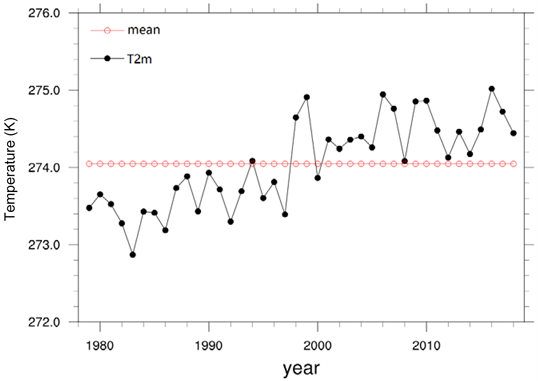Figure 2. Annual average temperature [K] of the Tibetan Plateau from 1979 to 2018 time series chart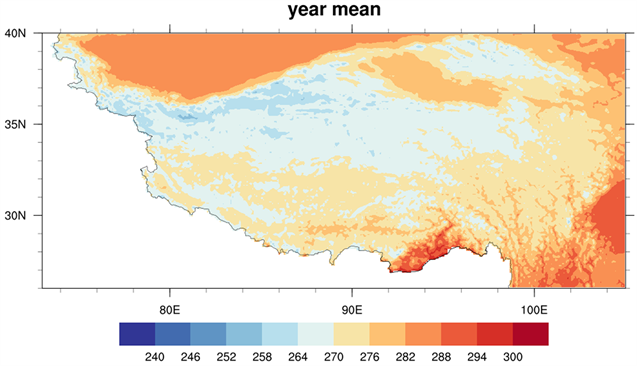Figure 3. Spatial distribution of mean temperature (K) on the Tibetan Plateau from 1979 to 2018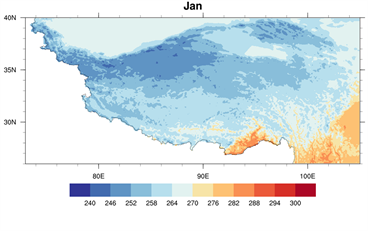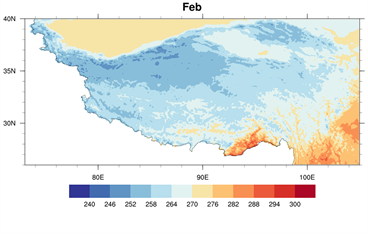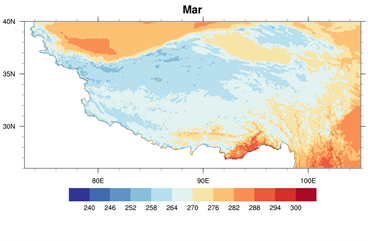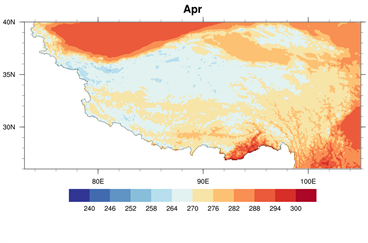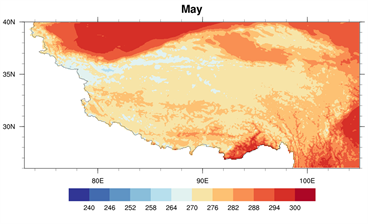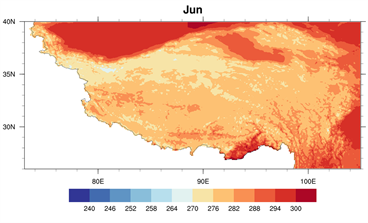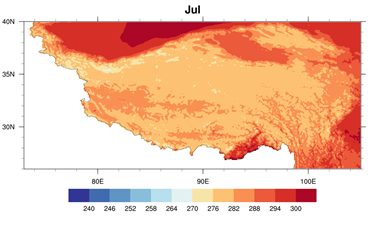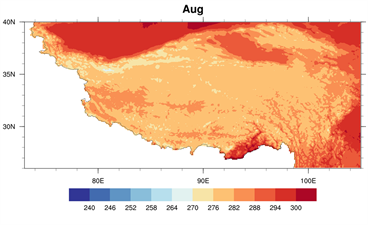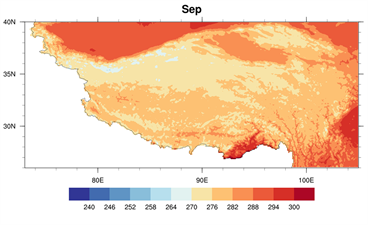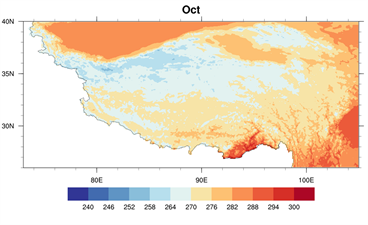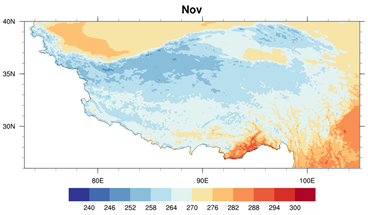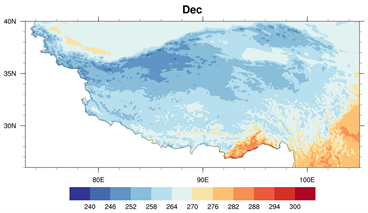Figure 4. Spatial distribution of monthly mean temperature (K) on the Tibetan Plateau from 1979 to 2018

3.1.2. 青藏高原地面两米处比湿时空分布特征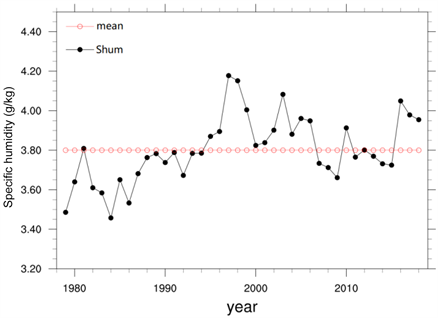Figure 5. Time series of the annual mean specific humidity (g/kg) of the Tibetan Plateau from 1979 to 2018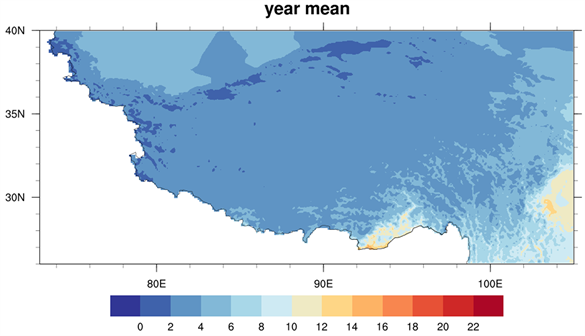Figure 6. Spatial distribution of annual average specific humidity (g/kg) on the Tibetan Plateau from 1979 to 2018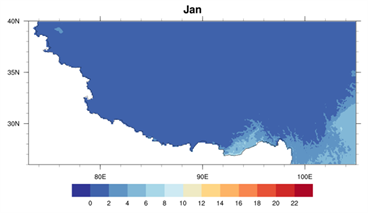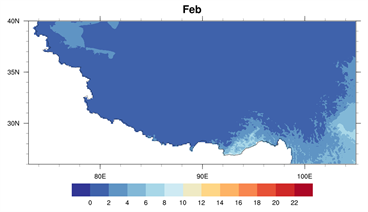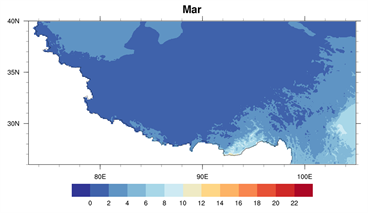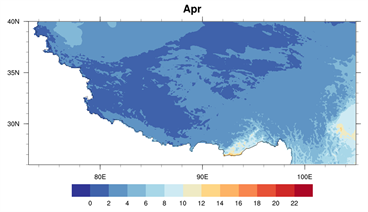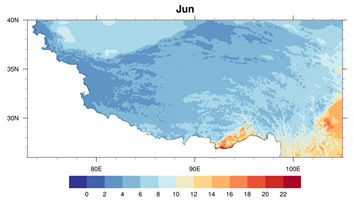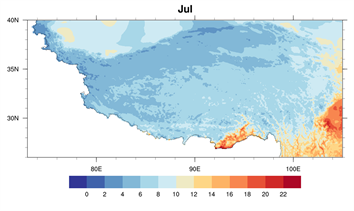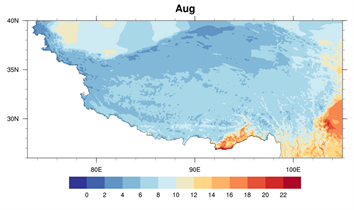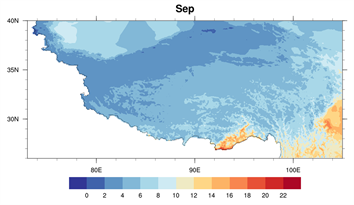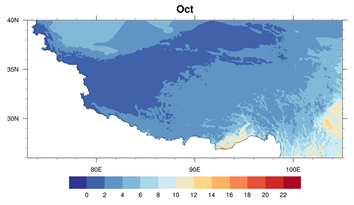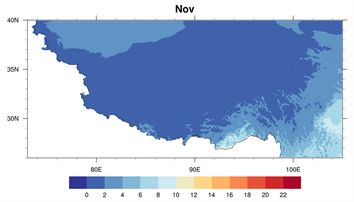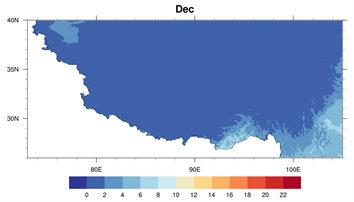Figure 7. Spatial distribution of monthly average specific humidity (g/kg) of the Qinghai-Tibet Plateau from 1979 to 2018

3.1.3. 青藏高原气温和湿度的关系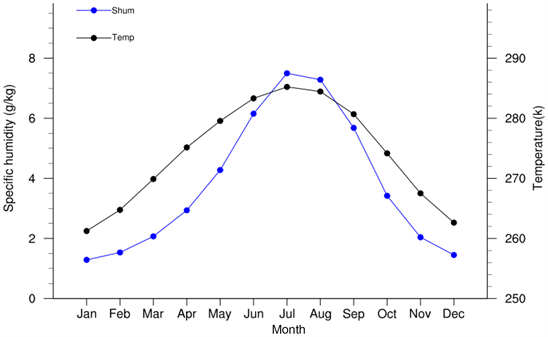Figure 8. Time series of the average annual temperature (K) and specific humidity (g/kg) from 1979 to 2018

3.2. 影响温度与湿度的气象因素特征分析Table 1. Correlation coefficients between selected meteorological elements and the examination of correlation coefficientsFigure 9. Time series of surface long wave radiation (W/m2) and annual average temperature (K) from 1979 to 2018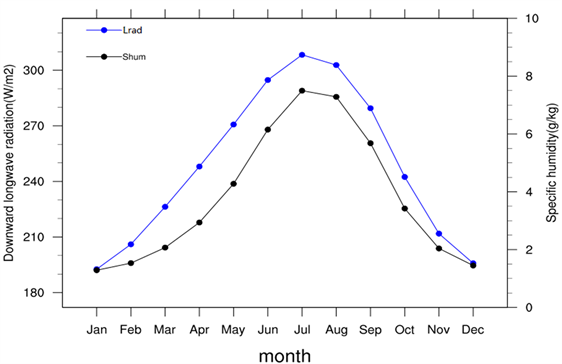Figure 10. Time series of surface long wave radiation (W/m2) and monthly average specific humidity (g/kg) from 1979 to 2018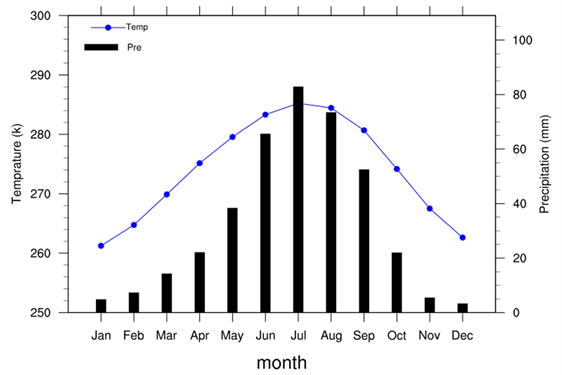Figure 11. Time series of monthly mean temperature (K) and monthly cumulative precipitation (mm) on the Tibetan Plateau from 1979 to 2018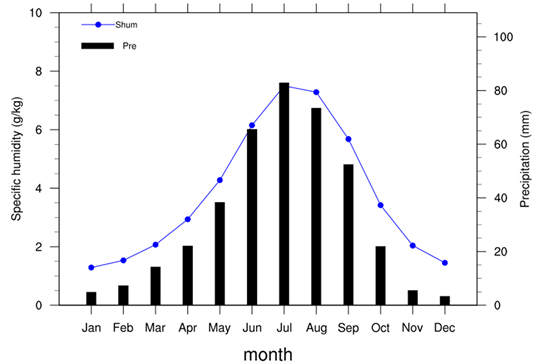Figure 12. Time series of monthly mean specific humidity (g/kg) and cumulative monthly precipitation (mm) of the Tibetan Plateau from 1979 to 2018

4. 结论

1) 气温与湿度在时间序列上的变化具有一致性，但湿度的变化幅度比气温更大；在1979~2018年年平均的空间分布上，气温分布与高原的地形分布有较大的相似性，而湿度的空间分布则与纬度有更大的关系；

2) 在高原区域中海拔高的藏北高原是高原的气温与湿度的低值区，海拔低的柴达木盆地则是温度的相对高值区；东南部的雅鲁藏布江流域与横断山脉由于地形是河谷的原因，是温度和湿度的高值区；

3) 年平均气温和比湿在径向平均和纬向平均下都与海拔高度有很大的负相关性，即气温和湿度都随海拔高度的增高而减小；

4) 气温和湿度除了受海拔高度和经纬度等自然因素的影响，还与向下长波辐射和降水这些气象要素有很大的相关性。

Temperature and Humidity Characteristics of the Qinghai-Tibet Plateau from 1979 to 2018[J]. 气候变化研究快报, 2020, 09(04): 340-354. https://doi.org/10.12677/CCRL.2020.94038

1. 1. IPCC (2007) Summary for Policymakers of Climate Change 2007: The Physical Science Basis.

2. 2. 梁玲, 李跃清, 胡豪然 等. 青藏高原夏季感热异常与川渝地区降水关系的数值模拟[J]. 高原气象, 2013, 32(6): 1538-1545.

3. 3. 刘晓东. 青藏高原隆升对亚洲季风形成和全球气候与环境变化的影响[J]. 高原气象, 1999, 18(3): 321-331.

4. 4. 周俊前, 刘新, 李伟平, 等. 青藏高原春季地表感热异常对西北地区东部降水变化的影响[J]. 高原气象, 2016, 35(4): 845-853.

5. 5. 王绍武, 叶瑾琳, 龚道溢, 等. 近百年中国年气温序列的建立[J]. 应用气象学报, 1998, 9(4): 392-401.

6. 6. 姚莉, 吴庆梅. 青藏高原气候变化特征[J]. 气象科技, 2002(3): 36-37+16.

7. 7. Liu, X. and Chen, B. (2000) Climatic Warming in the Tibetan Plateau during Recent Decades. International Journal of Climatology, 20, 1729-1742. https://doi.org/10.1002/1097-0088(20001130)20:14<1729::AID-JOC556>3.0.CO;2-Y

8. 8. You, Q., Kang, S., Pepin, N., et al. (2010) Relationship between Temperature Trend Magnitude, Elevation and Mean Temperature in the Tibetan Plateau from Homogenized Surface Stations and Reanalysis Data. Global and Planetary Change, 71, 124-133. https://doi.org/10.1016/j.gloplacha.2010.01.020

9. 9. 宋辞, 裴韬, 周成虎. 1960年以来青藏高原气温变化研究进展[J]. 地理科学进展, 2012, 31(11): 1503-1509.

10. 10. Tao, J., Zhang, Y., Zhu, J., et al. (2013) Eleva-tion-Dependent Temperature Change in the Qinghai-Xizang Plateau Grass Land during the Past Decade. Theoretical and Applied Climatology, 117, 61-71. https://doi.org/10.1007/s00704-013-0976-z

11. 11. Wu, S.H., Yin, Y.H., Du, Z., et al. (2007) Climatic Trends over the Tibetan Plateau during 1971-2000. Journal of Geographical Sciences, 17, 141-151. https://doi.org/10.1007/s11442-007-0141-7

12. 12. You, Q.L., Min, J.Z., Lin, H.B., et al. (2015) Observed Climatol-ogy and Trend in Relative Humidity in the Central and Eastern Tibetan Plateau. Journal of Geophysical Research Atmos-phere, 120, 3610-3621. https://doi.org/10.1002/2014JD023031

13. 13. Hardwick, J.R., Westra, S. and Sharma, A. (2011) Observed Rela-tionships between Extreme Sub-Daily Precipitation, Surface Temperature, and Relative Humidity. Geophysical Research Letters, 37, L22805. https://doi.org/10.1029/2010GL045081

14. 14. Simmons, A.J., Willett, K.M., Jones, P.D., et al. (2010) Low-Frequency Variations in Surface Atmospheric Humidity, Temperature, and Precipitation: Inferences from Reanal-yses and Monthly Gridded Observational Datasets. Journal of Geophysical Research-Atmospheres, l15, 1-21. https://doi.org/10.1029/2009JD012442